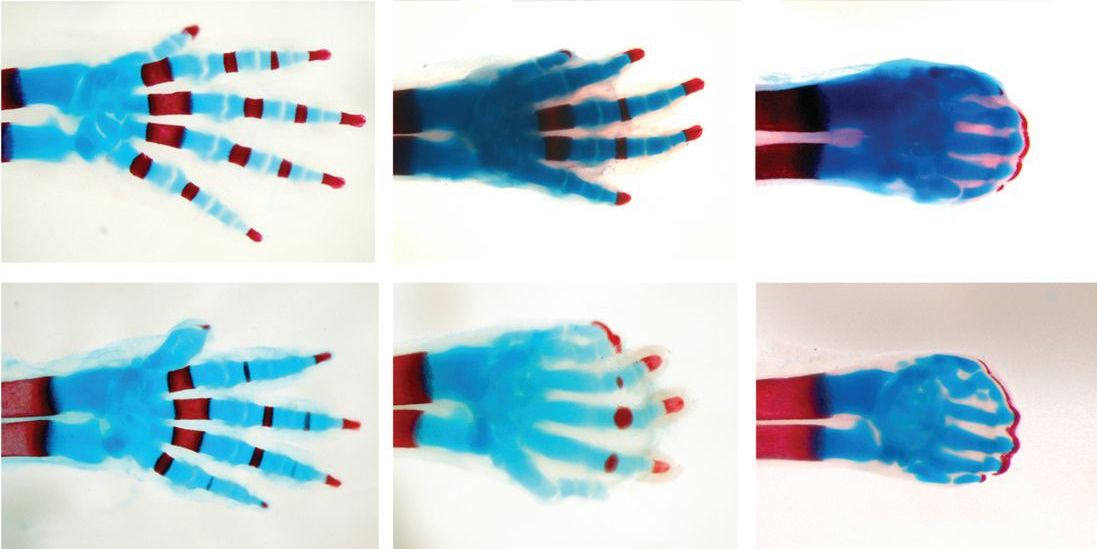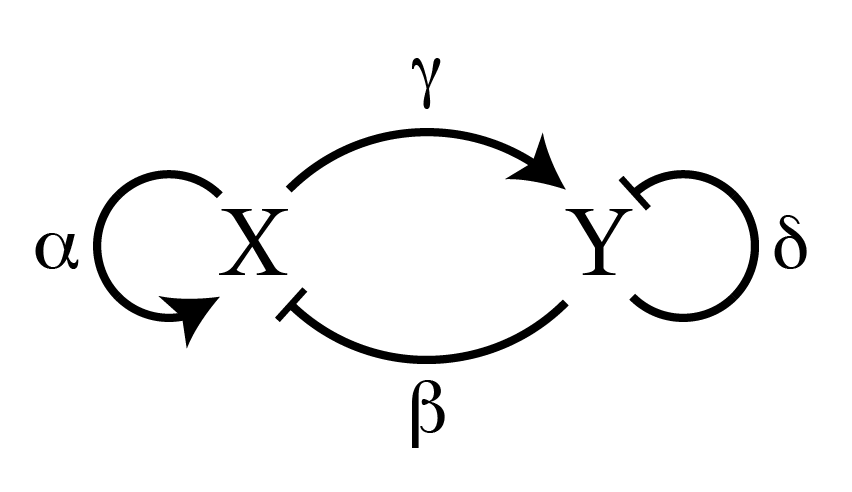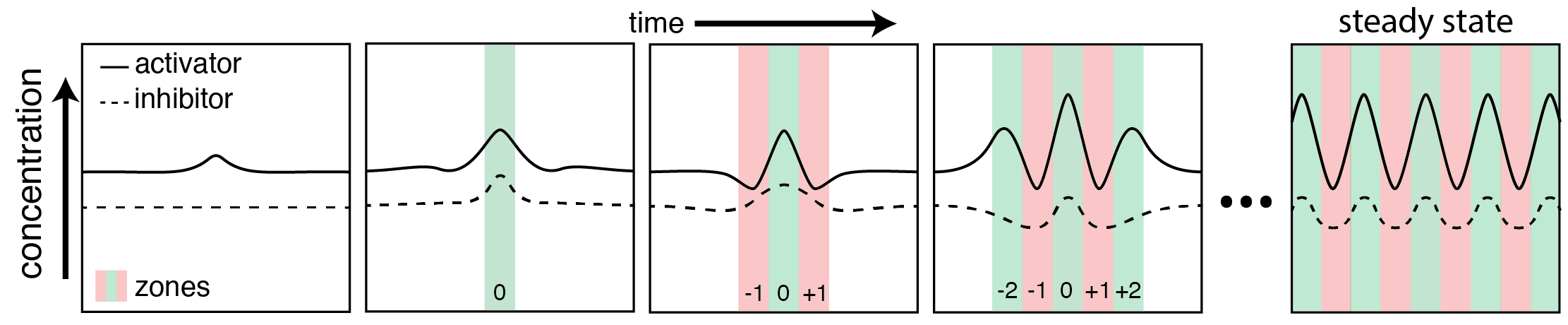# How the leopard got its spots

The human brain has evolved an arguably overactive habit of finding patterns. Who hasn’t spent an afternoon morphing clouds into various shapes? Similarly, groups of stars are transformed into bears, ladles, and warriors by ancient mystics and modern stargazers alike. Pattern recognition becomes more obviously useful looking at living things, where recognizing the difference between a harmless garter snake and a deadly asp is a skill worth having. We identify zebras by their stripes and leopards by their spots. But biology doesn’t just use external patterns. Organisms use internal, self-generated patterns to guide body formation. During the formation of a mouse’s paw, what will eventually become fingers are blueprinted by the certain proteins. Having the correct amount of each protein is critical to the correct number of the shape of fingers that are created  (Figure 1).Figure 1: The formation of fingers in the foot of a mouse requires a careful patterning of different proteins found during mouse development. If the amount of these proteins is changed, so does the number of eventual fingers. From Sheth et al. Science (2012)

In the mouse, these patterns are formed partly by genetic expression and partly by physical interactions between proteins. In today’s paper, we look at one of the first explanations of these physical interactions, given by the great mathematician Alan Turing. In his classic 1952 paper, The chemical basis of morphogenesis, Turing provides a simple explanation for how chemicals, like proteins, can interact with each other under known laws of physics to create striking patterns from a blank, uniform canvas.

Turing creates a pair of equations describing the rate of change of two different chemical species, X and Y, that he calls morphogens. The type of equations that Turing established is called reaction-diffusion equations. To understand Turing’s conclusions, I’ll first describe what is meant by reaction and diffusion and then put these concepts together to give a physical picture of how they can produce stripes. (See the appendix for a more detailed, mathematical description of the same phenomenon).

The reaction part of a reaction-diffusion equation describes the interactions between X and Y. A generic pair of coupled reaction equations will look like:$(\partial X / \partial t)_{reaction} = \alpha X + \beta Y$$(\partial Y / \partial t)_{reaction} = \gamma X + \delta Y$

Let’s look at just the first equation. On the left-hand side is the time derivative of the concentration of X, which measures how X changes in time depending on what is on the right-hand side. On the right-hand side, we see that the change in X over time is dependent on both itself and Y, multiplied by constant coefficients, alpha (?) and beta (?).

? tells us how strongly X affects itself, while ? tell us how strongly Y affects X. If ? is positive, we call Y an activator of X. If ? is negative, we call Y an inhibitor of X (similarly the sign of ? tells us whether X activates or inhibits itself, and the variables gamma (?) and delta (?) describe the behavior of how X and Y affect Y). We then can play around with different combinations of signs for all these coefficients to see how the system evolves in time.

The diffusion part of the reaction-diffusion equations describes how morphogens will move, on average, when they aren’t evenly distributed in space. The morphogens will move from areas of high concentration to areas of low concentration until everything is even, the same way a puff of perfume spreads across a room. The main difference between two morphogens is how quickly they move from high concentration to low concentration, which is quantified by a diffusion constant, D. If D is large, morphogens move rapidly, and if D is small, morphogens move slowly.

Turing found that a stable pattern can arise if we have a fast inhibitor and a slow activator. To be concrete, let’s call X the slow activator and Y the fast inhibitor. This means that DX<DY, and ?,?>0 and ?,?<0 in the reaction equations shown above. Therefore more X creates more of both X and Y, and more Y destroys more of both X and Y (Figure 2).Figure 2: Reaction network for X and Y in the activator-inhibitor model. The sign of the coefficients that couple the ?X/?t or ?Y/?t to X or Y determine what kind of arrow is used in the diagram. A positive coupling (activation) is shown with a pointed end (?), while a negative coupling (inhibition) is shown with a flat end (?).

Let’s start with a 1 dimensional, uniform distribution of both X and Y everywhere. Wherever Y exists, both X and Y get destroyed, and wherever X exists, both X and Y get created. If the distribution of X and Y are exactly equal, and they create and destroy at the same rates, then nothing will change. In reality, there will be very small variations over space of both X and Y. These small variations will lead to slightly more X or Y in particular spots (Figure 3).

Where more X exists, there will be an overall creation of both X and Y. Since X moves around more slowly, it will stay in the same spot for longer, while Y will quickly move away. The lingering X will produce even more X and Y, creating a region of excess X.  Y has then moved to neighboring areas and represses the production of both X and Y. However, eventually the concentration of Y decreases enough for the production properties of X to dominate again, and the whole system repeats itself. Thus, a static wave pattern is created from an initially (almost) uniform distribution (Figure 3).Figure 3: Schematic of pattern formation using reaction diffusion equations. First, a small shift away from zone 0 in the activator (X) occurs. This then leads to an increased amount of both the activator (X) and the inhibitor (Y) in zone 0. The inhibitor diffuses outwards faster than the activator. This diminishes the activator in zones ±1. Within zones ±1, the inhibitor also inhibits itself, leading to new regions, zones ±2, with an excess of activator again. Eventually, the system reaches a steady state: a wave pattern that no longer changes in time.

That gets at the gist of Turing’s argument. It truly is an amazing discovery because diffusion plays such an important role. Usually, diffusion is considered a process that “smooths” things. As stated above, it tends to move things from regions of high concentration to low concentration, until the same concentration is left everywhere. Here, it’s doing the exact opposite. Diffusion is helping create pockets of very high concentrations of morphogens. Turing’s genius was to recognize that diffusion could do this at all. It’s not only an equalizer, it can create order from chaos.

The wave described above is an example of what can happen in one dimension. The pattern evolves until a wave is set up, and then stops changing. With slight tweaks in the parameters put in the equations, more interesting patterns — ones that never stop changing in time — are also possible. Even more striking is the role that the size and shape of the area you consider has on the resulting patterns. Depending on the starting parameters, a number of different patterns can emerge, such as labyrinths, stripes, and spots (Figure 4).

Today, Turing’s model serves as the foundation for more realistic models of pattern formation. There hasn’t been much evidence for the exact activator-inhibitor mechanism that Turing has suggested, but by adding more than two chemicals or adjusting the parameters that Turing put forth, reaction-diffusion models have been used to help explain everything from the patterns on cat fur to the number of fingers on mouse paws.  Turing’s model shows that mathematical analysis is a valid way to explain some of the most striking and complex biological phenomena seen in nature.

 We can make this arbitrary choice because, for right now, X and Y are just labels. As long as one is a fast inhibitor and one is a slow activator, we get to describe them with whatever label we want.

 The reason for this is statistics. Think of flipping 500 coins. You know that you should expect 250 heads and 250 tails, but the probability of getting exactly that outcome is incredibly small (more likely you’ll be close, 248 heads and 252 tails, or 240 heads and 260 tails). For this reason, we may expect the concentrations of X and Y to both be exactly equal, but in reality, there will be some small difference between the two.

Appendix

A more mathematical explanation of Turing patterns can be found here